# Logical Functions

The category of functions known as the logical functions contains a strange hodgepodge of things. Chapter 8 discussed two of them: the If and Case conditional functions. The logical functions covered here include several that are new to FileMaker Pro 8.

The Let Function

The Let function enables you to simplify complex calculations by declaring variables to represent subexpressions. These variables exist only within the scope of the formula and can't be referenced in other places. As an example, this is a formula presented in Chapter 8 for extracting the last line of a text field:

`Right(myText; Length(myText) - Position(myText; "¶"; 1; PatternCount(myText; "¶")))`

With the Let function, this formula could be rewritten this way:

```Let ([fieldLength = Length(myText) ;
returnCount = PatternCount(myText; "¶") ;
positionOfLastReturn = Position (myText; "¶"; 1; returnCount) ;
charactersToGrab = fieldLength - positionOfLastReturn];
Right (myText, charactersToGrab)
)```

The Let function takes two parameters. The first is a list of variable declarations. If you want to declare multiple variables, you need to enclose the list within square brackets and separate the individual declarations within the list with semicolons. The second parameter is some formula you want evaluated. That formula can reference any of the variables declared in the first parameter, just as it would reference any field value.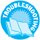If you experience unexpected behavior of a Let function, the trouble might be your variable names. For more information, see "Naming Variables in Let Functions" in the "Troubleshooting" section at the end of this chapter.

Notice in this example that the third variable declared, positionOfLastReturn, references the returnCount variable, which was the second variable declared. This capability to have subsequent variables reference previously defined ones is one of the powerful aspects of the Let function because it enables you to build up a complex formula via a series of simpler ones.

It is fair to observe that the Let function is never necessary; you could rewrite any formula that uses the Let function, without using Let, either as a complex nested formula or by explicitly defining or setting fields to contain subexpressions. The main benefits of using the Let function are simplicity, clarity, and ease of maintenance. For instance, a formula that returns a person's age expressed as a number of years, months, and days could be written as shown here:

```
```

[View full width]

Year (Get (CurrentDate)) - Year(birthDate) - (DayOfYear(Get(CurrentDate)) < DayOfYear(birthDate)) & " years, " & Mod ( Month(Get(CurrentDate)) - Month (birthDate) - (Day (Get(CurrentDate)) < Day(birthDate)); 12) & " months, and " & (Get(CurrentDate) - Date (Month(Get(CurrentDate)) - (Day (Get(CurrentDate)) < Day(birthDate)); Day (birthDate); Year (Get(CurrentDate)))) & " days "

This is a fairly complex nested formula, and many subexpressions appear multiple times. Writing and debugging this formula is difficult, even when you understand the logic on which it's based. With the Let function, the formula could be rewritten this way:

Let ( [ C = Get(CurrentDate); yC = Year (C) ; mC = Month (C) ; dC = Day (C) ; doyC = DayOfYear (C) ; B = birthDate; yB = Year (B) ; mB = Month (B) ; dB= Day (B) ; doyB = DayOfYear (b) ; num_years = ( yC - yB - (doyC < doyB)) ; num_months = Mod (mC - mB - (dC

Because of the extra space we've put in the formula, it's a bit longer than the original, but it's vastly easier to comprehend. If you were a developer needing to review and understand a formula written by someone else, we're sure you'd agree that you'd prefer seeing the Let version of this rather than the first version.

Besides simplicity and clarity, there are also performance benefits to using the Let function. If you have a complex subexpression that you refer to multiple times during the course of a calculation, FileMaker Pro evaluates it anew each time it's referenced. If you create the subexpression as a variable within a Let statement, the subexpression is evaluated only once, no matter how many times it is subsequently referenced. In the example just shown, for instance, FileMaker would evaluate Get(CurrentDate) eight times in the first version. In the version that uses Let, it's evaluated only once. In many cases, the performance difference may be trivial or imperceptible. But other times, optimizing the evaluation of calculation formulas may be just the answer for increasing your solution's performance.

The more you use the Let function, the more likely it is that it will become one of the core functions you use. To help you become more familiar with it, we use it frequently throughout the examples in the rest of this chapter.

## Quick Calculation Testing Using Let

The Let function makes it much easier to debug calculation formulas. It used to be that if you wanted to make sure that a subexpression was evaluating correctly, you'd need to create a separate field to investigate it. Using Let, you can just comment out the second parameter of the Let function and have the function return one or more of the subexpressions directly. When you've got each subexpression working as intended, just comment out the test code and uncomment the original code.

Tip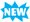It's not uncommon that you may want to set the same variable several times within a Let statement. A typical example occurs when you want to perform a similar operation several times on the same variable, without excessive nesting. For example, in FileMaker 7, a fragment of a Let statement that's involved in some complex text parsing might look like this:
``` result = _TextColor( text; RGB( 255: 0; 0 ));
result1 = _TextFont ( result; "TimesNewRoman");
result2 = _Textsize ( result1; 14);```

Here, we want to apply several text formatting operations to the value of text. We'd like to put them on successive rows, rather than building a big nested expression. We'd prefer to just keep naming the output result, but in FileMaker 7, we'll be prevented from setting a variable with the same name twice. In FileMaker 8 this behavior is permitted, and we could rewrite the code fragment as something like this:

``` result = _TextColor( text; RGB( 255: 0; 0 ));
result = _ TextFont ( result; "TimesNewRoman");
result = _Textsize ( result; 14);```

Although this is a great convenience when you need to do it, be aware that calculations and custom functions that use this technique will not execute correctly if the file is accessed via FileMaker Pro 7.

The Choose Function

The If and Case functions are sufficiently robust and elegant for most conditional tests that you'll write. For several types of conditional tests, however, the Choose function is a more appropriate option. As with If and Case, the value returned by the Choose function is dependent on the result of some test. What makes the Choose function different is that the test should return an integer rather than a true/false result. The test is followed by a number of possible results. The one that's chosen depends on the numeric result of the test. If the test result is 0, the first result is used. If the test result is 1, the second result is used, and so on. The syntax for Choose is as follows:

`Choose (test ; result if test=0 ; result if test=1 ; result if test=2 ....)`

A classic example of when a Choose function comes in handy is when you have categorical data stored as a number and you need to represent it as text. For instance, you might import demographic data in which the ethnicity of an individual is represented by an integer from 1 to 5. The following formula might be used to represent it to users:

```
```

[View full width]

Choose (EthnicityCode; ""; "African American"; "Asian"; "Caucasian"; "Hispanic"; " NativeAmerican")

Of course, the same result could be achieved with the following formula:

```
```

[View full width]

Case (EthnicityCode = 1; "African American"; EthnicityCode = 2; "Asian", EthnicityCode =3; "Caucasian"; EthnicityCode = 4; "Hispanic"; EthnicityCode= 5; "Native American")

You should consider the Choose function in several other situations. The first is for generating random categorical data. Say your third-grade class is doing research on famous presidents, and you want to randomly assign each student one of the six presidents you have chosen. By first generating a random number from 0 to 5, you can then use the Choose function to select a president. The formula would be this:

```Let ( r = Random * 6; // Generates a random number from 0 to 5
Choose (r, "Washington", "Jefferson", "Lincoln", "Roosevelt", "Truman", "Kennedy"))```

Don't worry that r isn't an integer; the Choose function ignores everything but the integer portion of a number.

Several FileMaker Pro functions return integer numbers from 1 to n, so these naturally work well as the test for a Choose function. Most notable are the DayofWeek function, which returns an integer from 1 to 7, and the Month function, which returns an integer from 1 to 12. As an example, you could use the Month function within a Choose to figure out within which quarter of the year a given date fell:

```
```

[View full width]

Choose (Month(myDate)-1; "Q1"; "Q1"; "Q1"; "Q2"; "Q2"; "Q2"; "Q3"; "Q3"; "Q3"; "Q4"; "Q4";"Q4")

The -1 shifts the range of the output from 112 to 011, which is more desirable because the Choose function is zero-based, meaning that the first result corresponds to a test value of zero. There are more compact ways of determining the calendar quarter of a date, but this version is very easy to understand and offers much flexibility.

Another example of when Choose works well is when you need to combine the results of some number of Boolean tests to produce a distinct result. As an example, imagine that you have a table that contains results on Myers-Briggs personality tests. For each test given, you have scores for four pairs of personality traits (E/I, S/N, T/F, J/P). Based on which score in each pair is higher, you want to classify each participant as one of 16 personality types. Using If or Case statements, you would need a very long, complex formula to do this. With Choose, you can treat the four tests as a binary number, and then simply do a conversion back to base-10 to decode the results. The formula might look something like this:

```Choose( (8 * (E>I)) + (4 * (S>N)) + (2 * (T>F)) + (J>P);
"Type 1 - INFP" ; "Type 2 - INFJ" ; "Type 3 - INTP" ; "Type 4 - INTJ" ;
"Type 5 - ISFP" ; "Type 6 - ISFJ" ; "Type 7 - ISTP" ; "Type 8 - ISTJ" ;
"Type 9 - ENFP" ; "Type 10 - ENFJ" ; "Type 11 - ENTP" ; "Type 12 - ENTJ" ;
"Type 13 - ESFP" ; "Type 14 - ESFJ" ; "Type 15 - ESTP" ; "Type 16 - ESTJ")```

Each greater-than comparison is evaluated as a 1 or 0 depending on whether it represents a true or false statement for the given record. By multiplying each result by successive powers of 2, you end up with an integer from 0 to 15 that represents each of the possible outcomes. (This is similar to how flipping a coin four times generates 16 possible outcomes.)

As a final example, the Choose function can also be used anytime you need to "decode" a set of abbreviations into their expanded versions. Take, for example, a situation in which survey respondents have entered SA, A, N, D, or SD as a response to indicate Strongly Agree, Agree, Neutral, Disagree, or Strongly Disagree. You could map from the abbreviation to the expanded text by using a Case function like this:

```Case (ResponseAbbreviation = "SA"; "Strongly Agree";
ResponseAbbreviation = "A"; "Agree" ;
ResponseAbbreviation = "N"; "Neutral" ;
ResponseAbbreviation = "D"; "Disagree" ;
ResponseAbbreviation = "SD"; "Strongly Disagree" )```

You can accomplish the same mapping by using a Choose function if you treat the two sets of choices as ordered lists. You simply find the position of an item in the abbreviation list, and then find the corresponding item from the expanded text list. The resulting formula would look like this:

```
```

[View full width]

Let ( [a = "|SA||A||N||D||SD|" ; r = "|" & ResponseAbbreviation & "|" ; pos = Position (a; r ; 1 ; 1) ; itemNumber = PatternCount (Left (a; pos-1); "|") / 2]; Choose (itemNumber, "Strongly Agree"; "Agree"; "Neutral"; "Disagree"; "StronglyDisagree") )

In most cases, you'll probably opt for using the Case function for simple decoding of abbreviations. Sometimes, however, the list of choices isn't something you can explicitly test against (such as with the contents of a value list), and finding one's position within the list may suffice to identify a parallel position in some other list. Having the Choose function in your toolbox may offer an elegant solution to such challenges.

The GetField Function

When writing calculation formulas, you use field names to refer abstractly to the contents of particular fields in the current record. That is, the formula for a FullName calculation might be FirstName & " " & LastName. FirstName and LastName are abstractions; they represent data contained in particular fields.

Imagine, however, that instead of knowing in advance what fields to refer to in the FullName calculation, you wanted to let users pick any fields they wanted to. So you set up two fields, which we'll call UserChoice1 and UserChoice2. How can you rewrite the FullName calculation so that it's not hard-coded to use FirstName and LastName, but rather uses the fields that users type in the two UserChoice fields?

The answer, of course, is the GetField function. GetField enables you to add another layer of abstraction to your calculation formulas. Instead of hard-coding field names in a formula, GetField allows you to place into a field the name of the field you're interested in accessing. That sounds much more complicated than it actually is. Using GetField, we might rewrite our FullName formula as shown here:

`GetField (UserChoice1) & " " & GetField (UserChoice2)`

The GetField function takes just one parameter. That parameter can be either a literal text string or a field name. Having it be a literal text string, although possible, is not particularly useful. The function GetField("FirstName") would certainly return the contents of the FirstName field, but you can achieve the same thing simply by using FirstName by itself. It's only when the parameter of the GetField function is a field or formula that it becomes interesting. In that case, the function returns the contents of the field referred to by the parameter.

There are many potential uses of GetField in a solution. Imagine, for instance, that you have a Contact table with fields called First Name, Nickname, and Last Name (among others). Sometimes contacts prefer to have their nickname appear on badges and in correspondence, and sometimes the first name is desired. To deal with this, you could create a new text field called Preferred Name and format that field as a radio button containing First Name and Nickname as the choices. When doing data entry, a user could simply check off which name should be used for correspondence. When it comes time to make a Full Name calculation field, one of your options would be the following:

```Case ( Preferred Name = "First Name"; First Name;
Preferred Name = "Nickname"; Nickname) &
" " & Last Name```

Another option, far more elegant and extensible, would be the following:

`GetField (PreferredName) & " " & Last Name`

When there are only two choices, the Case function certainly isn't cumbersome. But if there were dozens or hundreds of fields to choose from, GetField clearly has an advantage.

Building a Customizable List Report

One of the common uses of GetField is for building user-customizable list reports. It's really nothing more than an extension of the technique shown in the preceding example, but it's still worth looking at in depth. The idea is to have several global text fields where a user can select from a pop-up list of field names. The global text fields can be defined in any table you want. Remember, in calculation formulas, you can refer to a globally stored field from any table, even without creating a relationship to that table. The following example uses two tables: SalesPeople and Globals. The SalesPeople table has the following data fields:

SalesPersonID

FirstName

LastName

Territory

CommissionRate

Phone

Email

Sales_2005

Sales_2006

The Globals table has six global text fields named gCol1 through gCol6.

With these in place, you can now create six display fields in the SalesPeople table (named ColDisplay1 through ColDisplay6) that will contain the contents of the field referred to in one of the global fields. For instance, ColDisplay1 has the following formula:

`GetField (Globals::gCol1)`

ColDisplay2 through 6 will have similar definitions. The next step is to create a value list that contains all the fields you want the user to be able to select. The list used in this example is shown in Figure 14.1. Keep in mind that because the selection is used as part of a GetField function, the field names must appear exactly as they have been definedand any change to the underlying field names will cause the report to malfunction.

Figure 14.1. Define a value list containing a list of the fields from which you want to allow a user to select for the custom report.The final task is to create a layout where users can select and see the columns for their custom list report. You might want to set up one layout where the user selects the fields and another for displaying the results, but we think it's better to take advantage of the fact that in FileMaker 8, fields in header parts of list layouts can be edited. The column headers of your report can simply be pop-up lists. Figure 14.2 shows how you would set up your layout this way.

Figure 14.2. The layout for your customizable list report can be quite simple. Here, the selection fields act also as field headers.Back in Browse mode, users can now click into a column heading and select what data they want to appear there. This one layout can thus serve a wide variety of needs. Figures 14.3 and 14.4 show two examples of the types of reports that can be made.

Figure 14.3. A user can customize the contents of a report simply by selecting fields from pop-up lists in the header.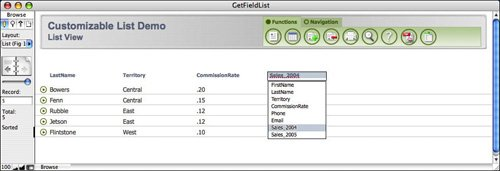Figure 14.4. Here's another example of a how a user might configure the customizable list report.Extending the Customizable List Report

After you have the simple custom report working, there are many ways you can extend it to add even more value and flexibility for your users. For instance, you might add a subsummary part that's also based on a user-specified field. A single layout can thus be a subsummary based on any field the user wants. One way to implement this is to add another pop-up list in the header of your report and a button to sort and preview the subsummary report. Figure 14.5 shows what your layout would look like after adding the subsummary part and pop-up list. BreakField is a calculation in the SalesPeople table that's defined as shown here:

`GetField (Globals::gSummarizeBy)`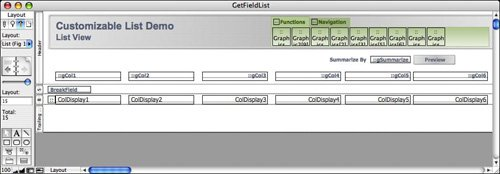The Preview button performs a script that sorts by the BreakField and goes to Preview mode. Figure 14.6 shows the result of running the script when Territory has been selected as the break field.

Figure 14.6. Sorting by the break field and previewing shows the results of the dynamic subsummary.Caution

To be fully dynamic, any calculations you write using the GetField function are probably going to need to be unstored. Unstored calculations will not perform well over very large data sets when searching and sorting, so use caution when creating GetField routines that might need to handle large data sets.

The Evaluate Function

The Evaluate function is one of the most intriguing functions in FileMaker Pro 8. In a nutshell, it enables you to evaluate a dynamically generated or user-generated calculation formula. With a few examples, you'll easily understand what this function does. It may, however, take a bit more time and thought to understand why you'd want to use it in a solution. We start with explaining the what, and then suggest a few potential whys.

The syntax for the Evaluate function is as follows:

`Evaluate ( expression {; [field1 ; field2 ;...]} )`

The expression parameter is a text string representing some calculation formula that you want evaluated. The optional additional parameter is a list of fields whose modification triggers the reevaluation of the expression.

For example, imagine that you have a text field named myFormula and another named myTrigger. You then define a new calculation field called Result, using the following formula:

`Evaluate (myFormula; myTrigger)`

Figure 14.7 shows some examples of what Result will contain for various entries in myFormula.

Figure 14.7. Using the Evaluate function, you can have a calculation field evaluate a formula contained in a field.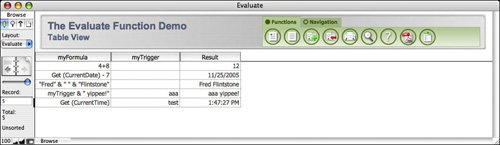There's something quite profound going on here. Instead of having to "hard-code" calculation formulas, you can evaluate a formula that's been entered as field data. In this way, Evaluate provides an additional level of logic abstraction similar to the GetField function. In fact, if myFormula contained the name of a field, Evaluate(myFormula) and GetField(myFormula) would return exactly the same result. It might help to think of Evaluate as the big brother of GetField. Whereas GetField can return the value of a dynamically specified field, Evaluate can return the value of a dynamically specified formula.

Uses for the Evaluate Function

A typical use for the Evaluate function is to track modification information about a particular field or fields. A timestamp field defined to auto-enter the modification time is triggered anytime any field in the record is modified. There may be times, however, when you want to know the last time that the Comments field was modified, without respect to other changes to the record. To do this, you would define a new calculation field called CommentsModTime with the following formula:

`Evaluate ("Get(CurrentTimestamp)" ; Comments)`

The quotes around Get(CurrentTimestamp) are important, and are apt be a source of confusion. The Evaluate function expects to be fed either a quote-enclosed text string (as shown here) or a formula that yields a text string (as in the Result field earlier). For instance, if you want to modify the CommentsModTime field so that rather than just returning a timestamp, it returns something like Record last modified at: 11/28/2005 12:23:58 PM by Fred Flintstone, you would need to modify the formula to the following:

```
```

[View full width]

Evaluate (""Record modified at: " & Get (CurrentTimeStamp) & " by " & Get(AccountName)" ; Comments)

Here, because the formula you want to evaluate contains quotation marks, you must escape them by preceding them with a slash. For a formula of any complexity, this becomes difficult both to write and to read. There is, fortunately, a function called Quote that eliminates all this complexity. The Quote function returns the parameter it is passed as a quote-wrapped text string, with all internal quotes properly escaped. Therefore, you could rewrite the preceding function more simply as this:

```
```

[View full width]

Evaluate (Quote ("Record modified at: " & Get (CurrentTimeStamp) & " by " & Get (AccountName)) ; Comments)

In this particular case, using the Let function further clarifies the syntax:

```Let ( [
time = Get ( CurrentTimeStamp ) ;
account = Get ( AccountName );
myExpression = Quote ( "Record modified at: " & time & " by " & account ) ] ;

Evaluate ( myExpression ; Comments )
)```

Evaluation Errors

You typically find two other functions used in conjunction with the Evaluate function: IsValidExpression and EvaluationError.

IsValidExpression takes as its parameter an expression, and it returns a 1 if the expression is valid, a 0 if it isn't. An invalid expression is any expression that can't be evaluated by FileMaker Pro, whether due to syntax errors or other runtime errors. If you plan to allow users to type calculation expressions into fields, be sure to use IsValidExpression to test their input to be sure it's well formed. In fact, you probably want to include a check of some kind within your Evaluate formula itself:

```Let ( valid = IsValidExpression (myFormula) ;
If (not valid; "Your expression was invalid" ; Evaluate (myFormula) )```

The EvaluationError function is likewise used to determine whether there's some problem with evaluating an expression. However, it returns the actual error code corresponding to the problem. One thing to keep in mind, however, is that rather than testing the expression, you want to test the evaluation of the expression. So, as an error trap used in conjunction with an Evaluate function, you might have the following:

```Let ( [result = Evaluate (myFormula) ;
error = EvaluationError (result) ] ;
If (error ; "Error: " & error ; result)
)```

Customizable List Reports Redux

We mentioned previously that Evaluate could be thought of as an extension of GetField. In an example presented in the GetField section, we showed how you could use the GetField function to create user-customizable report layouts. One of the drawbacks of that method that we didn't discuss at the time is that your field names need to be user- and display-friendly. However, there is an interesting way to get around this limitation that also happens to showcase the Evaluate function. We discuss that solution here as a final example of Evaluate.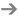Another use of Evaluate is presented in "Passing Multivalued Parameters," p. 438.

To recap the earlier example, imagine that you have six global text fields (gCol1 through gCol6) in a table called Globals. Another table, called SalesPeople, has demographic and sales-related data for your salespeople. Six calculation fields in SalesPeople, called ColDisplay1 through ColDisplay6, display the contents of the demographic or sales data fields, based on a user's selection from a pop-up list containing field names. ColDisplay1, for instance, has the following formula:

`GetField (Globals::gCol1)`

We now extend this solution in several ways. First, create a new table in the solution called FieldNames with the following text fields: FieldName and DisplayName. Figure 14.8 shows the data that might be entered in this table.

Figure 14.8. The data in FieldName represents fields in the SalesPerson table; the DisplayName field shows more user-friendly labels that will stand in for the actual field labels.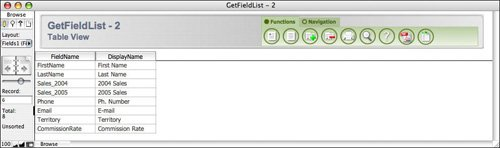Earlier, we suggested using a hard-coded value list for the pop-up lists attached to the column selection fields. Now you'll want to change that value list so that it contains all the items in the DisplayName column of the FieldNames table. Doing this, of course, causes all the ColDisplay fields to malfunction. There is, for instance, no field called Ph. Number, so GetField ("Ph. Number") will not function properly. What we want now is the GetField function not to operate on the user's entry, but rather on the FieldName that corresponds to the user's DisplayName selection. That is, when the user selects Ph. Number in gCol1, ColDisplay1 should display the contents of the Phone field.

You can accomplish this result by creating a relationship from the user's selection over to the DisplayName field. Because there are six user selection fields, there need to be six relationships. This requires that you create six occurrences of the FieldNames table. Figure 14.9 shows the Relationships Graph after you have set up the six relationships. The six new table occurrences are named Fields1 through Fields6. Notice that there's also a cross-join relationship between SalesPeople and Globals. This relationship allows you to look from SalesPeople all the way over to the FieldNames table.

Figure 14.9. To create six relationships from the Globals table to the FieldNames table, you need to create six occurrences of FieldNames.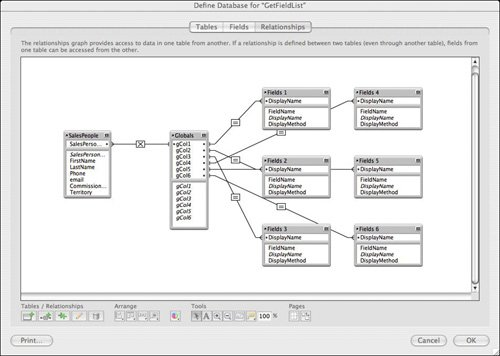The final step is to alter the calculation formulas in the ColDisplay fields. Remember, instead of "getting" the field specified by the user, we now want to get the field related to the field label specified by the user. At first thought, you might be tempted to redefine ColDisplay1 this way:

`GetField (Fields1::FieldName)`

The problem with this is that the only way that ColDisplay1 updates is if the FieldName field changes. Changing gCol1 doesn't have any effect on it. This, finally, is where Evaluate comes in. To force ColDisplay1 to update, you can use the Evaluate function instead of GetField. The second parameter of the formula can reference gCol1, thus triggering the reevaluation of the expression every time gCol1 changes. The new formula for ColDisplay1 is therefore this:

`Evaluate (Fields1::FieldName ; Globals::gCol1)`

There is, in fact, still a slight problem with this formula. Even though the calculation is unstored, the field values don't refresh onscreen. The solution is to refer not merely to the related FieldName, but rather to use a Lookup function (which is covered in depth in the next section) to explicitly grab the contents of FieldName. The final formula, therefore, is the following:

`Evaluate (Lookup (Fields1::FieldName) ; Globals::gCol1)`

There's one final interesting extension we will make to this technique. At this point, the Evaluate function is used simply to grab the contents of a field. It's quite possible, however, to add a field called Formula to the FieldNames table, and have the Evaluate function return the results of some formula that you define there. The formula in ColDisplay1 would simply be changed to this:

`Evaluate (Lookup (Fields1::Formula) ; Globals::gCol1)`

One reason you might want to do this is to be able to add some text formatting to particular fields. For instance, you might want the Sales_2004 field displayed with a leading dollar sign. Because all the ColDisplay fields yield text results, you can't do this with ordinary field formatting. Instead, in the Formula field on the Sales_2004 record, you could type the following formula:

`"\$ " & Sales_2004`

There's no reason, of course, why a formula you write can't reference multiple fields. This means that you can invent new fields for users to reference simply by adding a new record to the FieldNames table. For example, you could invent a new column called Initials, defined this way:

`Left (FirstName; 1) & Left (LastName; 1)`

You could even invent a column called Percent Increase that calculates the percent sales increase from 2004 to 2005. This would be the formula for that:

`Round((Sales_2005 - Sales_2004) / Sales_2004 *100, 2) & " %"`

Figure 14.10 shows the contents of the FieldNames table. Note that for columns where you just want to retrieve the value of a field (for example, FirstName), the field name itself is the entire formula.

Figure 14.10. The expression in the Formula field is dynamically evaluated when a user selects a column in the customizable report.This technique is quite powerful. You can cook up new columns for the customizable report just by adding records to the FieldNames table. Figure 14.11 shows an example of a report that a user could create based on the formulas defined in FieldNames. Keep in mind that Initials, \$ Increase, and Percent Increase have not been defined as fields anywhere.

Figure 14.11. In the finished report, users can select from any of the columns defined in the FieldNames table, even those that don't explicitly exist as defined fields.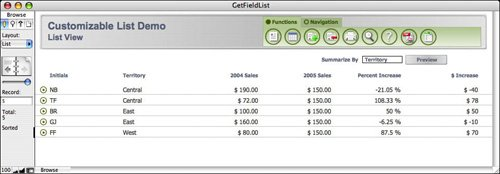The Lookup Functions

In versions of FileMaker before version 7, lookups were exclusively an auto-entry option. FileMaker 7 added two lookup functions, Lookup and LookupNext, and both are useful additions to any developer's toolkit.

The two lookup functions operate quite similarly to their cousin, the auto-entry lookup option. In essence, a lookup is used to copy a related value into the current table. Lookups (all kinds) have three necessary components: a relationship, a trigger field, and a target field. When the trigger field is modified, the target field is set to some related field value.

It's important to understand the functional differences between the lookup functions and the auto-entry option. Although they behave similarly, they're not quite equivalent. Some of the key differences include the following:

• Auto-entry of a looked-up value is an option for regular text, number, date, time, or timestamp fields, which are subsequently modifiable by the user. A calculation field that includes a lookup function is not user modifiable.
• The lookup functions can be used anywherenot just in field definitions. For instance, they can be used in formulas in scripts, record-level security settings, and calculated field validation. Auto-entering a looked-up value is limited to field definition.
• The lookup functions can be used in conjunction with other functions to create more complex logic rules. The auto-entry options are comparatively limited.

Lookup

The syntax of the Lookup function is as follows:

`Lookup ( sourceField {; failExpression} )`

The sourceField is the related field whose value you want to retrieve. The optional failExpression parameter is returned if there is no related record or if the sourceField is blank for the related record. If the specified relationship matches multiple related records, the value from the first related record is returned.

There are two main differences between using the Lookup function and simply referencing a related field in a formula. The first is that calculations that simply reference related fields must be unstored, but calculations that use the Lookup function to access related fields can be stored and indexed. The other difference is that changing the sourceField in the related table does not cause the Lookup to retrigger. Just as with auto-entry of a looked-up value, the Lookup function captures the sourceField as it existed at a moment in time. The alternative, simply referencing the related field, causes all the values to remain perfectly in sync: When the related value is updated, any calculations that reference it are updated as well. (The downside is that, as with all calculations that directly reference related data, such a calculation cannot be stored.)

LookupNext

The LookupNext function is designed to allow you to map continuous data elements to categorical results. It has the same effect as checking the Copy Next Lower Value or Copy Next Text Formatting Functions Higher Value options when specifying an auto-entry lookup field option. Here is its syntax:

`LookupNext ( sourceField ; lower/higherFlag )`

The acceptable values for the second parameter are Lower and Higher. These are keywords and shouldn't be placed in quotes.

An example should help clarify what we mean about mapping continuous data to categorical results. Imagine that you have a table that contains information about people, and that one of the fields is the person's birth date. You want to have some calculation fields that display the person's astrological information, such as a zodiac sign and ruling planet. Birth dates mapping to zodiac signs is a good example of continuous data mapping to categorical results: A range of birth dates corresponds to each zodiac sign.

In practice, two small but instructive complications arise when you try to look up zodiac signs. The first complication is that the zodiac date ranges are expressed not as full dates, but merely as months and days (for example, Cancer starts on June 22 regardless of what year it is). This means that when you set up your zodiac table, you'll use text fields rather than date fields for the start and end dates. The second complication is that Capricorn wraps around the end of the year. The easiest way to deal with this is to have two records in the Zodiac table for Capricorn, one that spans December 22December 31, and the other that spans January 1January 20.

Figure 14.12 shows the full data of the Zodiac table. The StartDate and EndDate fields, remember, are actually text fields. The leading zeros are important for proper sorting.

Figure 14.12. The data from the Zodiac table is looked up and is transferred to a person record based on the person's birth date.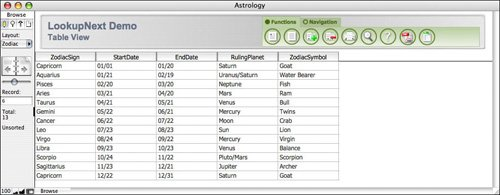In the Person table, you need to create a calculation formula that generates a text string containing the month and date of the person's birth date, complete with leading zeros so that it's consistent with the way dates are represented in the Zodiac table. The DateMatch field is defined this way:

`Right ("00" & Month (Birthdate); 2) & "/" & Right ("00"& Day (Birthdate); 2)`

Next, create a relationship between the Person and Zodiac tables, matching the DateMatch field in Person to the StartDate field in Zodiac. This relationship is shown in Figure 14.13.

Figure 14.13. By relating the Person table to Zodiac, you can look up any information you want based on the person's birth date.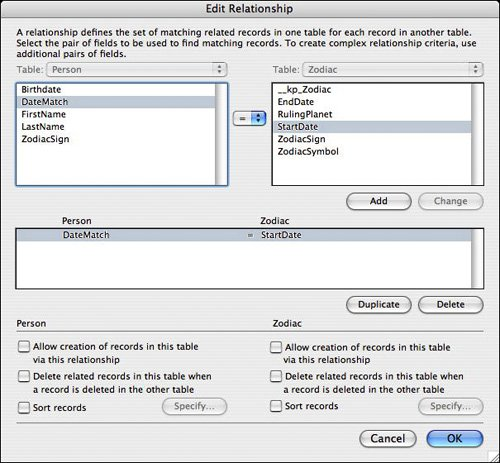Obviously, many birth dates aren't start dates for one of the zodiac signs. To match to the correct zodiac record, you want to find the next lower match when no exact match is found. For instance, with a birth date of February 13 (02/13), there is no matching record where the StartDate is 02/13, so the next lowest StartDate, which is 01/21 (Aquarius), should be used.

In the Person table, therefore, you can grab any desired zodiac information by using the LookupNext function. Figure 14.14 shows an example of how this date might be displayed on a person record. The formula for ZodiacInfo is as follows:

```"Sign: " & LookupNext (Zodiac::ZodiacSign; Lower) & "¶" &
"Symbol: " & LookupNext (Zodiac::ZodiacSymbol; Lower) & "¶" &
"Ruling Planet: " & LookupNext (Zodiac::RulingPlanet; Lower)```

Figure 14.14. Using the LookupNext function, you can create a calculation field in the Person table that contains information from the next lower matching record.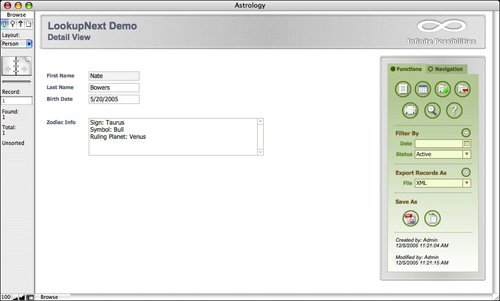It would have been possible in the previous examples to match to the EndDate instead of the StartDate. In that case, you would simply need to match to the next higher instead of the next lower matching record.

An entirely different but perfectly valid way of approaching the problem would have been to define a more complex relationship between Person and Zodiac, in which the DateMatch was greater than or equal to the StartDate and less than or equal to the EndDate. Doing this would allow you to use the fields from the Zodiac table as plain related fields; no lookup would have been required. There are no clear advantages or disadvantages of this method over the one discussed previously.

Note

Other typical scenarios for using LookupNext are for things such as shipping rates based on weight ranges, price discounts based on quantity ranges, and defining cut scores based on continuous test score ranges.

### Text Formatting Functions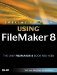Special Edition Using FileMaker 8
ISBN: 0789735121
EAN: 2147483647
Year: 2007
Pages: 296